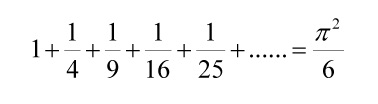# This Educative Video Presents a Proof of the Basel Problem Using Light

3Blue1Brown have created a wonderful video that will widen your math knowledge.

This wonderfully educative video from 3Blue1Brown describes a geometric answer to the Basel problem. The Basel problem asks for the ‘precise summation of the reciprocals of the squares of the natural numbers, i.e. the precise sum of the infinite series...'It was first posed by Pietro Mengoli in 1644 and solved by Leonhard Euler in 1734. Because Euler was from Basel, the mathematical problem is named after his home city.

The problem asks for the exact sum of this series as well as a proof that this sum is correct. Euler found the exact sum to be π2/6 and announced this discovery in 1735.

However, it wasn’t until 1741 that he was able to produce a truly rigorous proof to justify his discovery. The host of 3Blue1Brown, Grant Sanderson, uses a different way to prove the sum. In this case, he offers a geometric answer.

The video uses light as a way to describe this complex mathematical problem. You’ll need some basic math education to fully get the gist of this video, but once you have got your definitions sorted the video is so well explained, even a beginner mathematician will be able to gain an understanding of the math theory presented here.